# Multiplicative inverse

Explain why the reciprocal of - 1 1/17 is the multiplicative inverse of - 17/18.

i =  -0.9444

### Step-by-step explanation: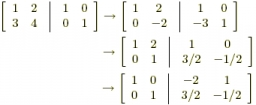Did you find an error or inaccuracy? Feel free to write us. Thank you!Tips to related online calculators
Need help to calculate sum, simplify or multiply fractions? Try our fraction calculator.

## Related math problems and questions:

• Reciprocal equation 3Solve reciprocal equation: 1/2 + 2/3=1/x
• Reciprocal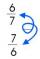Calculate the reciprocal numbers for the given real numbers.
• Sign of a expressionWhat is the sign of expression: minus 18 start fraction 9 divided by 17 end fraction plus left parenthesis minus 18 start fraction 9 divided by 17 end fraction right parenthesis?
• EmberEmber spent $144 on her birthday party for 18 guests. If the cost is at the same rate, how many guests will there if she spends$176?
• Lorry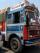The lorry was loaded with 18 boxes of 15 kg. How many boxes with weight 18 kg can be loaded, if total load must be same?
• How many 15How many six-eights are there in 18? Which of the following would you choose to solve this question? 18 x 6/8 or 18 : 6/8
• PoolIf water flows into the pool by two inlets, fill the whole for 19 hours. The first inlet filled pool 5 hour longer than the second. How long pool take to fill with two inlets separately?
• Eq with reciprocalSolve given equation with reciprocal member: a-6/a+10=4/8
• Olda wanted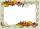Olda wanted to enlarge the 9 cm x 13 cm photo so that the shorter side measured 18 cm. How big will the longer side of the photo be?
• ŽSRCalculate fixed annual personnel costs of operating monorail line 118 km long if every 5 km is a station, which serves three people - one dispatcher and two switchmen in 4-shift operation. Consider the average salary of the employee 885 €.
• Metallurgical enterprise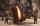Metallurgical company daily produces 318 tons of cast iron. How many tons of coke it burns to melt cast iron when 10 tons of cast consumes 6 tons of coke?
• To improper fractionChange mixed number to improper fraction a) 1 2/15 b) -2 15/17
• Sum of 18Sum of two fractions is 4 3/7. If one of the fractions is 2 1/5 find the other one .
• Reciprocal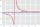It is true (prove it) that if a> b> 0: ?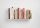If I read 15 pages a day, I will read the whole book in 18 days. How long will it take to read a book if I only read 9 pages every day?630g of rice is needed to prepare mushroom risotto for four people. How many boxes of 500g rice do you have to buy to prepare risotto for 17 people?Determine the number of all positive integers less than 4183444 if each is divisible by 29, 7, 17. What is its sum?